Prealgebra 2e

# 10.2Use Multiplication Properties of Exponents

Prealgebra 2e10.2 Use Multiplication Properties of Exponents

### Learning Objectives

By the end of this section, you will be able to:

• Simplify expressions with exponents
• Simplify expressions using the Product Property of Exponents
• Simplify expressions using the Power Property of Exponents
• Simplify expressions using the Product to a Power Property
• Simplify expressions by applying several properties
• Multiply monomials

### Be Prepared 10.4

Before you get started, take this readiness quiz.

Simplify: $34·34.34·34.$
If you missed the problem, review Example 4.25.

### Be Prepared 10.5

Simplify: $(−2)(−2)(−2).(−2)(−2)(−2).$
If you missed the problem, review Example 3.52.

### Simplify Expressions with Exponents

Remember that an exponent indicates repeated multiplication of the same quantity. For example, $2424$ means to multiply four factors of $2,2,$ so $2424$ means $2·2·2·2.2·2·2·2.$ This format is known as exponential notation.

### Exponential NotationThis is read $aa$ to the $mthmth$ power.

In the expression $am,am,$ the exponent tells us how many times we use the base $aa$ as a factor.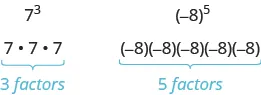Before we begin working with variable expressions containing exponents, let’s simplify a few expressions involving only numbers.

### Example 10.11

Simplify:

1. $5353$
2. $9191$

### Try It 10.21

Simplify:

1. $4343$
2. $111111$

### Try It 10.22

Simplify:

1. $3434$
2. $211211$

### Example 10.12

Simplify:

1. $(78)2(78)2$
2. $(0.74)2(0.74)2$

### Try It 10.23

Simplify:

1. $(58)2(58)2$
2. $(0.67)2(0.67)2$

### Try It 10.24

Simplify:

1. $(25)3(25)3$
2. $(0.127)2(0.127)2$

### Example 10.13

Simplify:

1. $(−3)4(−3)4$
2. $−34−34$

### Try It 10.25

Simplify:

1. $(−2)4(−2)4$
2. $−24−24$

### Try It 10.26

Simplify:

1. $(−8)2(−8)2$
2. $−82−82$

### Simplify Expressions Using the Product Property of Exponents

You have seen that when you combine like terms by adding and subtracting, you need to have the same base with the same exponent. But when you multiply and divide, the exponents may be different, and sometimes the bases may be different, too. We’ll derive the properties of exponents by looking for patterns in several examples. All the exponent properties hold true for any real numbers, but right now we will only use whole number exponents.

First, we will look at an example that leads to the Product Property.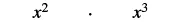What does this mean? How many factors altogether?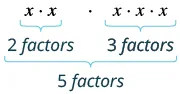So, we haveNotice that 5 is the sum of the exponents, 2 and 3.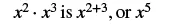We write: $x2⋅x3x2⋅x3$$x2+3x2+3$$x5x5$

The base stayed the same and we added the exponents. This leads to the Product Property for Exponents.

### Product Property of Exponents

If $aa$ is a real number and $m,nm,n$ are counting numbers, then

$am·an=am+nam·an=am+n$

To multiply with like bases, add the exponents.

An example with numbers helps to verify this property.

$22·23=?22+34·8=?2532=32✓22·23=?22+34·8=?2532=32✓$

### Example 10.14

Simplify: $x5·x7.x5·x7.$

### Try It 10.27

Simplify: $x7·x8.x7·x8.$

### Try It 10.28

Simplify: $x5·x11.x5·x11.$

### Example 10.15

Simplify: $b4·b.b4·b.$

### Try It 10.29

Simplify: $p9·p.p9·p.$

### Try It 10.30

Simplify: $m·m7.m·m7.$

### Example 10.16

Simplify: $27·29.27·29.$

### Try It 10.31

Simplify: $6·69.6·69.$

### Try It 10.32

Simplify: $96·99.96·99.$

### Example 10.17

Simplify: $y17·y23.y17·y23.$

### Try It 10.33

Simplify: $y24·y19.y24·y19.$

### Try It 10.34

Simplify: $z15·z24.z15·z24.$

We can extend the Product Property of Exponents to more than two factors.

### Example 10.18

Simplify: $x3·x4·x2.x3·x4·x2.$

### Try It 10.35

Simplify: $x7·x5·x9.x7·x5·x9.$

### Try It 10.36

Simplify: $y3·y8·y4.y3·y8·y4.$

### Simplify Expressions Using the Power Property of Exponents

Now let’s look at an exponential expression that contains a power raised to a power. See if you can discover a general property.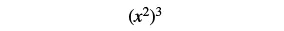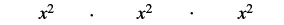What does this mean? How many factors altogether?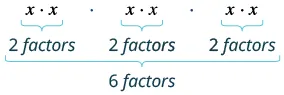So, we have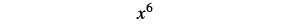Notice that 6 is the product of the exponents, 2 and 3.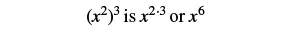We write: $(x2)3(x2)3$$x2⋅3x2⋅3$$x6x6$

We multiplied the exponents. This leads to the Power Property for Exponents.

### Power Property of Exponents

If $aa$ is a real number and $m,nm,n$ are whole numbers, then

$(am)n=am·n(am)n=am·n$

To raise a power to a power, multiply the exponents.

An example with numbers helps to verify this property.

$(52)3=?52·3(25)3=?5615,625=15,625✓(52)3=?52·3(25)3=?5615,625=15,625✓$

### Example 10.19

Simplify:

1. $(x5)7(x5)7$
2. $(36)8(36)8$

### Try It 10.37

Simplify:

1. $(x7)4(x7)4$
2. $(74)8(74)8$

### Try It 10.38

Simplify:

1. $(x6)9(x6)9$
2. $(86)7(86)7$

### Simplify Expressions Using the Product to a Power Property

We will now look at an expression containing a product that is raised to a power. Look for a pattern.

 $(2x)3(2x)3$ What does this mean? $2x·2x·2x2x·2x·2x$ We group the like factors together. $2·2·2·x·x·x2·2·2·x·x·x$ How many factors of 2 and of $x?x?$ $23·x323·x3$ Notice that each factor was raised to the power. $(2x)3is23·x3(2x)3is23·x3$ We write: $(2x)3(2x)3$ $23·x323·x3$

The exponent applies to each of the factors. This leads to the Product to a Power Property for Exponents.

### Product to a Power Property of Exponents

If $aa$ and $bb$ are real numbers and $mm$ is a whole number, then

$(ab)m=ambm(ab)m=ambm$

To raise a product to a power, raise each factor to that power.

An example with numbers helps to verify this property:

$(2·3)2=?22·3262=?4·936=36✓(2·3)2=?22·3262=?4·936=36✓$

### Example 10.20

Simplify: $(−11x)2.(−11x)2.$

### Try It 10.39

Simplify: $(−14x)2.(−14x)2.$

### Try It 10.40

Simplify: $(−12a)2.(−12a)2.$

### Example 10.21

Simplify: $(3xy)3.(3xy)3.$

### Try It 10.41

Simplify: $(−4xy)4.(−4xy)4.$

### Try It 10.42

Simplify: $(6xy)3.(6xy)3.$

### Simplify Expressions by Applying Several Properties

We now have three properties for multiplying expressions with exponents. Let’s summarize them and then we’ll do some examples that use more than one of the properties.

### Properties of Exponents

If $a,ba,b$ are real numbers and $m,nm,n$ are whole numbers, then

$Product Propertyam·an=am+nPower Property(am)n=am·nProduct to a Power Property(ab)m=ambmProduct Propertyam·an=am+nPower Property(am)n=am·nProduct to a Power Property(ab)m=ambm$

### Example 10.22

Simplify: $(x2)6(x5)4.(x2)6(x5)4.$

### Try It 10.43

Simplify: $(x4)3(x7)4.(x4)3(x7)4.$

### Try It 10.44

Simplify: $(y9)2(y8)3.(y9)2(y8)3.$

### Example 10.23

Simplify: $(−7x3y4)2.(−7x3y4)2.$

### Try It 10.45

Simplify: $(−8x4y7)3.(−8x4y7)3.$

### Try It 10.46

Simplify: $(−3a5b6)4.(−3a5b6)4.$

### Example 10.24

Simplify: $(6n)2(4n3).(6n)2(4n3).$

### Try It 10.47

Simplify: $(7n)2(2n12).(7n)2(2n12).$

### Try It 10.48

Simplify: $(4m)2(3m3).(4m)2(3m3).$

### Example 10.25

Simplify: $(3p2q)4(2pq2)3.(3p2q)4(2pq2)3.$

### Try It 10.49

Simplify: $(u3v2)5(4uv4)3.(u3v2)5(4uv4)3.$

### Try It 10.50

Simplify: $(5x2y3)2(3xy4)3.(5x2y3)2(3xy4)3.$

### Multiply Monomials

Since a monomial is an algebraic expression, we can use the properties for simplifying expressions with exponents to multiply the monomials.

### Example 10.26

Multiply: $(4x2)(−5x3).(4x2)(−5x3).$

### Try It 10.51

Multiply: $(7x7)(−8x4).(7x7)(−8x4).$

### Try It 10.52

Multiply: $(−9y4)(−6y5).(−9y4)(−6y5).$

### Example 10.27

Multiply: $(34c3d)(12cd2).(34c3d)(12cd2).$

### Try It 10.53

Multiply: $(45m4n3)(15mn3).(45m4n3)(15mn3).$

### Try It 10.54

Multiply: $(23p5q)(18p6q7).(23p5q)(18p6q7).$

### Section 10.2 Exercises

#### Practice Makes Perfect

Simplify Expressions with Exponents

In the following exercises, simplify each expression with exponents.

55.

$4 5 4 5$

56.

$10 3 10 3$

57.

$( 1 2 ) 2 ( 1 2 ) 2$

58.

$( 3 5 ) 2 ( 3 5 ) 2$

59.

$( 0.2 ) 3 ( 0.2 ) 3$

60.

$( 0.4 ) 3 ( 0.4 ) 3$

61.

$( −5 ) 4 ( −5 ) 4$

62.

$( −3 ) 5 ( −3 ) 5$

63.

$−5 4 −5 4$

64.

$−3 5 −3 5$

65.

$−10 4 −10 4$

66.

$−2 6 −2 6$

67.

$( − 2 3 ) 3 ( − 2 3 ) 3$

68.

$( − 1 4 ) 4 ( − 1 4 ) 4$

69.

$− 0.5 2 − 0.5 2$

70.

$− 0.1 4 − 0.1 4$

Simplify Expressions Using the Product Property of Exponents

In the following exercises, simplify each expression using the Product Property of Exponents.

71.

$x 3 · x 6 x 3 · x 6$

72.

$m 4 · m 2 m 4 · m 2$

73.

$a · a 4 a · a 4$

74.

$y 12 · y y 12 · y$

75.

$3 5 · 3 9 3 5 · 3 9$

76.

$5 10 · 5 6 5 10 · 5 6$

77.

$z · z 2 · z 3 z · z 2 · z 3$

78.

$a · a 3 · a 5 a · a 3 · a 5$

79.

$x a · x 2 x a · x 2$

80.

$y p · y 3 y p · y 3$

81.

$y a · y b y a · y b$

82.

$x p · x q x p · x q$

Simplify Expressions Using the Power Property of Exponents

In the following exercises, simplify each expression using the Power Property of Exponents.

83.

$( u 4 ) 2 ( u 4 ) 2$

84.

$( x 2 ) 7 ( x 2 ) 7$

85.

$( y 5 ) 4 ( y 5 ) 4$

86.

$( a 3 ) 2 ( a 3 ) 2$

87.

$( 10 2 ) 6 ( 10 2 ) 6$

88.

$( 2 8 ) 3 ( 2 8 ) 3$

89.

$( x 15 ) 6 ( x 15 ) 6$

90.

$( y 12 ) 8 ( y 12 ) 8$

91.

$( x 2 ) y ( x 2 ) y$

92.

$( y 3 ) x ( y 3 ) x$

93.

$( 5 x ) y ( 5 x ) y$

94.

$( 7 a ) b ( 7 a ) b$

Simplify Expressions Using the Product to a Power Property

In the following exercises, simplify each expression using the Product to a Power Property.

95.

$( 5 a ) 2 ( 5 a ) 2$

96.

$( 7 x ) 2 ( 7 x ) 2$

97.

$( − 6 m ) 3 ( − 6 m ) 3$

98.

$( − 9 n ) 3 ( − 9 n ) 3$

99.

$( 4 r s ) 2 ( 4 r s ) 2$

100.

$( 5 a b ) 3 ( 5 a b ) 3$

101.

$( 4 x y z ) 4 ( 4 x y z ) 4$

102.

$( − 5 a b c ) 3 ( − 5 a b c ) 3$

Simplify Expressions by Applying Several Properties

In the following exercises, simplify each expression.

103.

$( x 2 ) 4 · ( x 3 ) 2 ( x 2 ) 4 · ( x 3 ) 2$

104.

$( y 4 ) 3 · ( y 5 ) 2 ( y 4 ) 3 · ( y 5 ) 2$

105.

$( a 2 ) 6 · ( a 3 ) 8 ( a 2 ) 6 · ( a 3 ) 8$

106.

$( b 7 ) 5 · ( b 2 ) 6 ( b 7 ) 5 · ( b 2 ) 6$

107.

$( 3 x ) 2 ( 5 x ) ( 3 x ) 2 ( 5 x )$

108.

$( 2 y ) 3 ( 6 y ) ( 2 y ) 3 ( 6 y )$

109.

$( 5 a ) 2 ( 2 a ) 3 ( 5 a ) 2 ( 2 a ) 3$

110.

$( 4 b ) 2 ( 3 b ) 3 ( 4 b ) 2 ( 3 b ) 3$

111.

$( 2 m 6 ) 3 ( 2 m 6 ) 3$

112.

$( 3 y 2 ) 4 ( 3 y 2 ) 4$

113.

$( 10 x 2 y ) 3 ( 10 x 2 y ) 3$

114.

$( 2 m n 4 ) 5 ( 2 m n 4 ) 5$

115.

$( −2 a 3 b 2 ) 4 ( −2 a 3 b 2 ) 4$

116.

$( −10 u 2 v 4 ) 3 ( −10 u 2 v 4 ) 3$

117.

$( 2 3 x 2 y ) 3 ( 2 3 x 2 y ) 3$

118.

$( 7 9 p q 4 ) 2 ( 7 9 p q 4 ) 2$

119.

$( 8 a 3 ) 2 ( 2 a ) 4 ( 8 a 3 ) 2 ( 2 a ) 4$

120.

$( 5 r 2 ) 3 ( 3 r ) 2 ( 5 r 2 ) 3 ( 3 r ) 2$

121.

$( 10 p 4 ) 3 ( 5 p 6 ) 2 ( 10 p 4 ) 3 ( 5 p 6 ) 2$

122.

$( 4 x 3 ) 3 ( 2 x 5 ) 4 ( 4 x 3 ) 3 ( 2 x 5 ) 4$

123.

$( 1 2 x 2 y 3 ) 4 ( 4 x 5 y 3 ) 2 ( 1 2 x 2 y 3 ) 4 ( 4 x 5 y 3 ) 2$

124.

$( 1 3 m 3 n 2 ) 4 ( 9 m 8 n 3 ) 2 ( 1 3 m 3 n 2 ) 4 ( 9 m 8 n 3 ) 2$

125.

$( 3 m 2 n ) 2 ( 2 m n 5 ) 4 ( 3 m 2 n ) 2 ( 2 m n 5 ) 4$

126.

$( 2 p q 4 ) 3 ( 5 p 6 q ) 2 ( 2 p q 4 ) 3 ( 5 p 6 q ) 2$

Multiply Monomials

In the following exercises, multiply the following monomials.

127.

$( 12 x 2 ) ( −5 x 4 ) ( 12 x 2 ) ( −5 x 4 )$

128.

$( −10 y 3 ) ( 7 y 2 ) ( −10 y 3 ) ( 7 y 2 )$

129.

$( −8 u 6 ) ( −9 u ) ( −8 u 6 ) ( −9 u )$

130.

$( −6 c 4 ) ( −12 c ) ( −6 c 4 ) ( −12 c )$

131.

$( 1 5 r 8 ) ( 20 r 3 ) ( 1 5 r 8 ) ( 20 r 3 )$

132.

$( 1 4 a 5 ) ( 36 a 2 ) ( 1 4 a 5 ) ( 36 a 2 )$

133.

$( 4 a 3 b ) ( 9 a 2 b 6 ) ( 4 a 3 b ) ( 9 a 2 b 6 )$

134.

$( 6 m 4 n 3 ) ( 7 m n 5 ) ( 6 m 4 n 3 ) ( 7 m n 5 )$

135.

$( 4 7 x y 2 ) ( 14 x y 3 ) ( 4 7 x y 2 ) ( 14 x y 3 )$

136.

$( 5 8 u 3 v ) ( 24 u 5 v ) ( 5 8 u 3 v ) ( 24 u 5 v )$

137.

$( 2 3 x 2 y ) ( 3 4 x y 2 ) ( 2 3 x 2 y ) ( 3 4 x y 2 )$

138.

$( 3 5 m 3 n 2 ) ( 5 9 m 2 n 3 ) ( 3 5 m 3 n 2 ) ( 5 9 m 2 n 3 )$

#### Everyday Math

139.

Email Janet emails a joke to six of her friends and tells them to forward it to six of their friends, who forward it to six of their friends, and so on. The number of people who receive the email on the second round is $62,62,$ on the third round is $63,63,$ as shown in the table. How many people will receive the email on the eighth round? Simplify the expression to show the number of people who receive the email.

Round Number of people
$11$ $66$
$22$ $6262$
$33$ $6363$
$……$ $……$
$88$ $??$
140.

Salary Raul’s boss gives him a $5%5%$ raise every year on his birthday. This means that each year, Raul’s salary is $1.051.05$ times his last year’s salary. If his original salary was $40,00040,000$, his salary after $11$ year was $40,000(1.05),40,000(1.05),$ after $22$ years was $40,000(1.05)2,40,000(1.05)2,$ after $33$ years was $40,000(1.05)3,40,000(1.05)3,$ as shown in the table below. What will Raul’s salary be after $1010$ years? Simplify the expression, to show Raul’s salary in dollars.

Year Salary
$11$ $40,000(1.05)40,000(1.05)$
$22$ $40,000(1.05)240,000(1.05)2$
$33$ $40,000(1.05)340,000(1.05)3$
$……$ $……$
$1010$ $??$

#### Writing Exercises

141.

Use the Product Property for Exponents to explain why $x·x=x2.x·x=x2.$

142.

Explain why $−53=(−5)3−53=(−5)3$ but $−54≠(−5)4.−54≠(−5)4.$

143.

Jorge thinks $(12)2(12)2$ is $1.1.$ What is wrong with his reasoning?

144.

Explain why $x3·x5x3·x5$ is $x8,x8,$ and not $x15.x15.$

#### Self Check

After completing the exercises, use this checklist to evaluate your mastery of the objectives of this section.

After reviewing this checklist, what will you do to become confident for all objectives?

Order a print copy

As an Amazon Associate we earn from qualifying purchases.